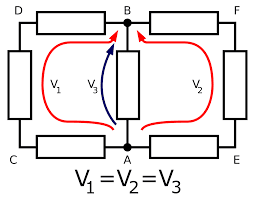Kirchoff's Circuit Rules - Kirchhoff's Circuit Laws - Electrical C & V

# Electrical C & V

electrical engineering information, basic electrical, electrical laws, machines, transformer, motors, types of motor, induction motor, dc motor, servo motor, synchronous motor, dynamo or generator, power system, transmission line, mcc, generation station, electronics, devices, diodes, sensors, circuit breaker, miniature circuit breaker, air circuit breaker, vaccum circuit breaker, sf6 circuit breaker, variable frequency drive

## 29 March 2020

Kirchoff's Circuit Rules - Kirchhoff's Circuit Laws

Sometimes using Ohm's law to find the value of voltage or current in a complex circuit creates problems.
In 1845, a German physicist named Gustav Kirchhoff proposed two rules regarding voltage and current to solve these problems in electrical circuits. Both these rules are called Kirchhoff's Circuit Laws. Like Ohm's law, Kirchhoff's laws have great importance in the field of electrical engineering.

The two rules of the circuit that Kirchoff stated were:

1. Kirchhoff's current law (KCL)
2. Kirchhoff's voltage law (KVL)

1. Kirchhoff's current law (KCL)

Definition of Kirchhoff's current law: "The algebraic sum of currents found on any point or junction or node in an electrical circuit will be zero."Kirchhoff's Current laws

In other words - "The sum of currents coming at a joint or a junction (where more than two conductors meet) in the electrical circuits is equal to the sum of the currents going from there."

This is also called Kirchhoff's 'Junction rule'. It is based on the principle of conservation of charge.

Example: According to the diagram, it can be understood by the following equation-

i1 + i2 - i3 - i4 - i5 ​​= 0

Or, i1 + i2 = i3 + i4 + i5

2. Kirchhoff's voltage law

Definition of Kirchhoff's voltage law - "The algebraic sum of the electric carrying force (EMF) in a closed loop or circuit is equal to the algebraic sum of the voltages generated at the ends of the resistors of that circuit.Kirchoff's volltage law

In other words, - "The algebraic sum of all the voltages of a loop is zero."

This is also called Kirchhoff's 'loop rule'. It is based on the law of conservation of energy.

Example: According to the diagram, it can be understood by the following equation-

V1 + V2 + V3 = 0

Some terminology

1. Node: The node, the junction of a node, where two or more conductors or any other element of the electrical circuit meets and is called a node.

2. Loop: A loop is a closed circuit in which the elements or nodes related to the circuit are not more than one.

3. Circuit: A circuit is a closed loop in which current flows through drivers, resistors, etc.